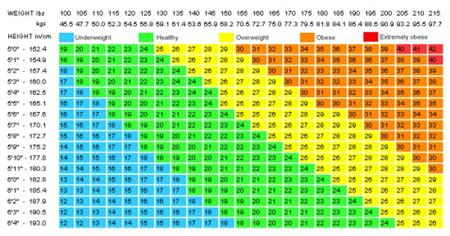Jan
19
2011

# Who Invented Body Mass Index

Body mass Index or BMI is a measure of body weight of a person in reference to his height. It tries to explain what should be the actual weight-height ratio of a person and follows an easy method for calculation. It is generally used for calculating whether a person is overweight, ideal weight or underweight. On a large scale, BMI is used to analyze composition of the population of an area, region or country. It was first invented by Adolphe Quetelet, a poly-mathematician somewhere in between 1835-1850.

The formula as invented by Quetelet was body mass in kg’s divided by the square of the height in meters. In 1995, the World Health Organization has introduced a common scale to decide whether a person is underweight or overweight. A person having a BMI of 18.5 or less is considered underweight whereas a person exceeding 25 is considered as overweight. For a score above 30, the person is said to be obese. Most countries follow the BMI scale as decided by W.H.O; however the countries like Japan and Singapore follow their own set of BMI scale. BMI has been used in many medical fields, statistical analysis of data for many decades due to its simplicity in calculation.